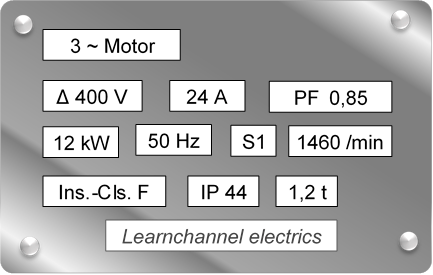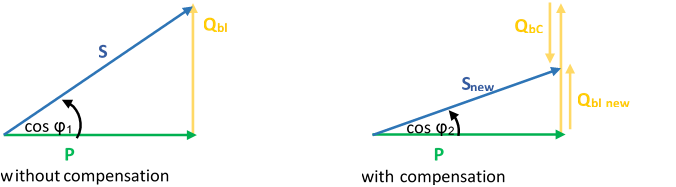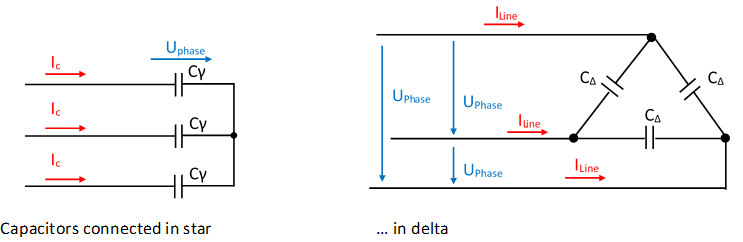## Compensation of three-phase asynchronous motors

Why compensate?

Because large inductive loads strain the power supply system, It is recommended that big induction motors should not be compensated.
Therefore, capacitors are added to improve the power factor PF or cos φ.

As a reference value motors above 5 kvar should be compensated. Calculate the reactive power consumption in rated operation for the motor shown below and decide whether this motor must be compensated or not.Motor plate 3 Phase Induction motor

Ql = √3 U * I * sin φ    | auxiliary calculation: PF = cos φ = 0,85   => φ ≈ 31,7888   => sin φ ≈ 0,52678

Ql = √3 * 400V * 24A * 0,52678 = 8,763 kvar    =>   The motor should be compensated.

In practice, you will not compensate all the reactive power that occurs at nominal load. The reason is: At low load (the extreme case would be no-load), lower reactive currents will flow and you would have overcompensated the motor, which is undesirable.
Either a target power factor is specified or the capacitor power can be taken from a table.

According to the specification the motor should be compensated at cos φ2 = 0.98. The power triangle with and without compensation you can take from the following sketch:Power vector diagram

Note:
active power P  in kW
apparent power S in kVA
reactive power in kvar

Determine the required capacitive reactive power Qbc to get the new active power factor:

Qc ges = Pzu (tan φ1  -  tan φ2 |  φ1  before compensation;   φ2  after compensation

In our case:
P = √3 U * I * cos φ  = √3 * 400V * 24A * 0,85  = 14,133 kW

Before compensation: cos φ1 = 0,85  =>  φ1 ≈ 31,79°
After compensation: cos φ2 = 0,98  => φ2 ≈ 11,48°
=>  Qc total = 14,133 kW (tan 31,79° - tan11,48°) = 5,889 kvar

Determinate the capacity of each capacitor:
First of all, it is possible to connect the capacitors in star or delta:Capacitors for compensation

The total reactive power of our motor is Qbc total = 5.889 kvar. Whether in star or delta, 1/3 of the reactive power now takes a single capacitor:
Qbc = 1/3 Qbc total = 1/3 * 5.889 kvar = 1.963 kvar
To show how the capacitive reactive resistance is related to the reactive power, we make a "bridge" to the ohmic resistance:

P = U2                    compared with       QC  =  U2                      … Eq. (1)
.      R                                                                    XC

and   XC  =  1 / 2π f C           … Eq. (2)

… Eq.(2) in …Eq.(1) results in:  QC =     U2         =   U2 2π f C
.                                                                   1 / 2π f C

=>       C =     QC      =    QC                 …Eq.(3)      | ω = 2π f
U2 2π f       U2 ω

From equation ...(3) it can be seen that tondetermine the capacitor size it is important to know whether they are connected in star or delta connection. Why? If the capacitors are connected in star, the capacitor voltage (phase voltage) is reduced by the factor √3 to 230 V (400 V line voltage), i.e. the capacitance of the capacitors is increased three times.
We check this statement:
Capacitors connected in delta:

C =     QC      =     1963 var             = 3,905 * 10-5 F  ≈  39 µF
U2 2π f      (400V)2 2π50s-1

Kondensatoren in Stern geschaltet:

C =     QC       =     1963 var              = 1,181 * 10-4 F  ≈  118 µF
.      U2 2π f        (230V)2 2π50s-1